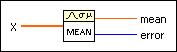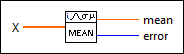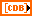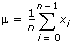# Mean VI

## LabVIEW 2018 Help

Edition Date: March 2018
Part Number: 371361R-01
View Product InfoLabVIEW 2016 HelpLabVIEW 2017 HelpLabVIEW 2018 HelpLabVIEW 2019 HelpLabVIEW 2020 Help

Owning Palette: Probability & Statistics VIs

Requires: Base Development System

Computes the mean of the values in the input sequence X. You must manually select the polymorphic instance you want to use.

Use the pull-down menu to select an instance of this VI.

 Select an instance Mean (DBL)Mean (CDB)

## Mean (DBL)X is the input sequence. If the input sequence X is empty, mean is NaN.mean is the mean, or average, of the values in the input sequence X.error returns any error or warning from the VI. You can wire error to the Error Cluster From Error Code VI to convert the error code or warning into an error cluster.

## Mean (CDB)X is the input sequence. If the input sequence X is empty, mean is NaN.mean is the mean, or average, of the values in the input sequence X.error returns any error or warning from the VI. You can wire error to the Error Cluster From Error Code VI to convert the error code or warning into an error cluster.

## Mean Details

The VI calculates mean using the following equation.where µ is mean and n is the number of elements in X.

## Example

Refer to the Statistics Solver VI in the labview\examples\Mathematics\Probability and Statistics directory for an example of using the Mean VI.